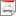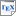[KNS01b] M. Kwiatkowska, G. Norman and J. Sproston. Symbolic computation of maximal probabilistic reachability. In K. Larsen and M. Nielsen (editors), Proc. 13th International Conference on Concurrency Theory (CONCUR'01), volume 2154 of LNCS, pages 169--183, Springer. August 2001. [ps.gz] [pdf] [bib] Downloads:ps.gz (100 KB)pdf (297 KB)bib Notes: The original publication is available at link.springer.com. Links: [Google] [Google Scholar] [CiteSeer] Abstract. We study the maximal reachability probability problem for infinite-state systems featuring both nondeterministic and probabilistic choice. The problem involves the computation of the maximal probability of reaching a given set of states, and underlies decision procedures for the automatic verification of probabilistic systems. We extend the framework of symbolic transition systems, which equips an infinite-state system with an algebra of symbolic operators on its state space, with a symbolic encoding of probabilistic transitions to obtain a model for an infinite-state probabilistic system called a symbolic probabilistic system. An exact answer to the maximal reachability probability problem for symbolic probabilistic systems is obtained algorithmically via iteration of a refined version of the classical predecessor operation, combined with intersection operations. As in the non-probabilistic case, our state space exploration algorithm is semi-decidable for infinite-state systems. We illustrate our approach with examples of probabilistic timed automata, for which previous approaches to this reachability problem were either based on unnecessarily fine subdivisions of the state space, or which obtained only an upper bound on the exact reachability probability.